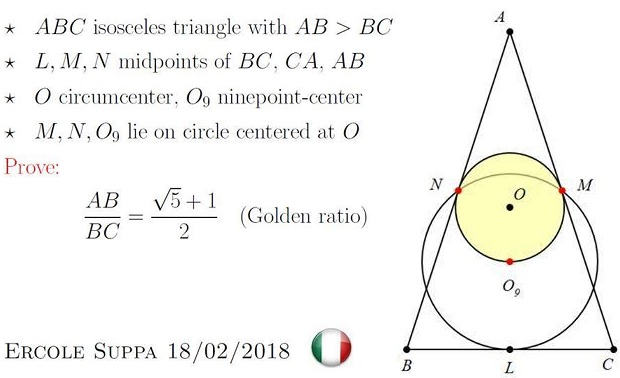# Golden Ratio in Isosceles Triangle II

### Source

This problem was posted by Ercole Suppa at the Peru Geometrico facebook group, and pointed to me by Leo Giugiuc. I post one solution below. More proofs can be found at the aforementioned link.### Problem

In an isosceles $\Delta ABC,$ $AB=AC\gt BC,$ circle $C(O,M,N)$ centered at the circumcenter $O$ and passing through the midpoints $M$ and $N$ of the side $AB$ and $AC,$ respectively also passes through $O_9$ the center of the nine-point circle. Let $r$ be the radius of $(MNO_9)$ and $r_9$ the radius of the nine-point circle $(LMN).$Prove that $\displaystyle\frac{AC}{BC}=\frac{r_9}{r}=\varphi,$ the Golden Ratio.

### Solution

Let's denote $a=BC$ and $b=AC,$ and let $R$ be the circumradius of $\Delta ABC.$ Note that $R=2r_9.$

Triangles $AON$ and $ACL$ are similar, so that

$\displaystyle \frac{2r_9}{r}=\frac{R}{r}=\frac{AO}{ON}=\frac{AC}{CL}=\frac{b}{a/2}=\frac{2b}{a}.$

This proves $\displaystyle \frac{r_9}{r}=\frac{b}{a}.$ Let $t$ stand for that ratio.In $\Delta AON,$ $AO^2-ON^2=AN^2,$ i.e., $\displaystyle (2r_9)^2-r^2=\left(\frac{b}{2}\right)^2$.

In $\Delta OLC,$ $CO^2-OL^2=CL^2,$ i.e., $\displaystyle (2r_9)^2-(r+r_9)^2=\left(\frac{a}{2}\right)^2$.

Dividing the former by the latter, $\displaystyle \frac{4t^2-1}{3t^2-2t-1}=t^2,$ or

$3t^4-2t^3-5t^2+1=0.$

But $3t^4-2t^3-5t^2+1=(t^2-t-1)(3t^2+t-1).$ The only positive root of the equation $t^2-t-1=0$ is $t=\varphi,$ the Golden Ratio. The only positive root of the other factor $3t^2+t-1=0$ is $\displaystyle t=\frac{\sqrt{13}-1}{6}\lt\frac{4-1}{6}=\frac{1}{2},$ which could not be the ratio $\displaystyle \frac{b}{a}$ of the two sides of $\Delta ABC.$

### Extra

If $H$ is the orthocenter of $\Delta ABC,$ then $\displaystyle \frac{HO_9}{HL}=\varphi,$ the Golden ratio.### Golden Ratio